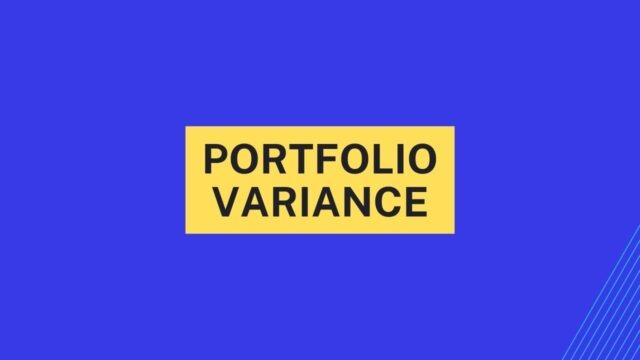# Portfolio Variance## What is portfolio variance and why should you care about it

Portfolio variance is a measure of the volatility of a portfolio, which is the degree to which the portfolio’s return fluctuates over time. investors care about portfolio variance because they want to know how much risk they are taking on when they invest in a particular portfolio. The higher the variance, the greater the risk. There are two types of risk that investors must take into account when considering an investment: systematic risk and unsystematic risk.

Systematic risk is the risk that cannot be diversified away, such as the risk of economic recession. Unsystematic risk is specific to a particular security or industry and can be diversified away. Investors usually seek to minimize unsystematic risk while still accepting some degree of systematic risk. Portfolio variance is one way to measure unsystematic risk. By looking at the variance of a portfolio, investors can get an idea of how much its return is likely to fluctuate and thereby how risky it is. Therefore, understanding variance is essential for any investor who wants to make informed investment decisions.

## Calculating portfolio variance

To calculate portfolio variance, investors first need to calculate the variance of each asset in the portfolio. To do this, they need to determine the mean return of each asset and then subtract that from each asset’s actual return. This gives them the asset’s return deviation. They then square each return deviation and add up all of the squared deviations. This sum is divided by the number of periods, which gives them the variance of each asset.

Once investors have calculated the variances of each asset, they need to calculate the covariance between each pair of assets. To do this, they multiply the return deviation of one asset by the return deviation of another asset and then take the average of all these products. This gives them the covariance between two assets.

To calculate portfolio variance, investors need to add up all of the variances of each asset and then add up all of the covariances between pairs of assets. This sum is then divided by the number of periods, which gives them the portfolio variance. Portfolio variance can be used to help investors understand how risky an investment portfolio is and make decisions about how to allocate their assets.

## Reducing portfolio variance

One way to reduce portfolio variance is to diversify investments. This means investing in a variety of different asset classes, such as stocks, bonds, and cash. By spreading out investments, it is possible to reduce the overall risk while still maintaining the potential for a high return. Another way to reduce portfolio variance is to invest in index funds. Index funds are a type of mutual fund that track a specific market index, such as the S&P 500. Because they are diversified and passively managed, index funds tend to have lower fees and expenses than other types of mutual funds. As a result, they offer investors a way to reduce portfolio variance without sacrificing potential returns.

## Examples of reducing portfolio variance

There are a number of ways to reduce portfolio variance. One is to invest in a variety of assets, such as stocks, bonds, and cash. This diversification will help to minimize the impact of any one investment on the overall portfolio. Another way to reduces variance is through asset allocation, which involves carefully balancing the mix of assets in the portfolio. This can be done by constructing a portfolio that is made up of investments with low correlations to one another. Finally, rebalancing the portfolio on a regular basis can also help to reduce variance. This involves selling assets that have appreciated in value and using the proceeds to purchase other assets that may be undervalued. By following these steps, investors can help to keep their portfolios well diversified and insulated from market volatility.

## How to apply what you’ve learned to your own investment portfolio

One of the most important things you can do when learning about investing is to apply what you’ve learned to your own portfolio. This will help you see how different investment strategies work in practice and allow you to make better decisions about where to put your money. There are a few different ways you can do this. First, you can create a mock portfolio using fake money. This will let you try out different investment strategies without putting any real money at risk.

Alternatively, you can start slowly by investing a small amount of money in a few different companies or funds. Once you’ve gained some experience, you can then increase your investment levels. By applying what you’ve learned to your own portfolio, you’ll be able to make wiser decisions about where to put your money and potentially earn higher returns.